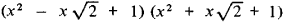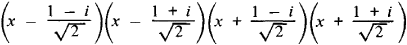# polynomial

(redirected from Polynomial systems of equations)
Also found in: Dictionary, Thesaurus, Medical.

## polynomial,

mathematical expression which is a finite sum, each term being a constant times a product of one or more variables raised to powers. With only one variable the general form of a polynomial is a0xn+a1xn−1+a2xn−2+…+an−1x+an where n is a positive integer and a0, a1, a2, … , an are any numbers. An example of a polynomial in one variable is 11x4−3x3+7x2+x−8. The degree of a polynomial in one variable is the highest power of the variable appearing with a nonzero coefficient; in the example given above, the degree is 4.

## Polynomial

an expression of the form

Axkytwm + Bxnupwq + … + Dxryswt

where x, y, … , w are variables, and A, B, … , D (coefficients of the polynomial) and k, l, … , t (exponents, which are nonnegative integers) are constants. Separate terms of the form Axkylwm are said to be terms of the polynomial. Both the order of the terms and the order of the factors in each term can vary arbitrarily. Terms with zero coefficients may be introduced or eliminated, and factors with zero exponents in each term may likewise be introduced or eliminated. A polynomial with one, two, or three terms is called a monomial, binomial, or trinomial, respectively. Two terms of a polynomial are said to be similar if the exponents of identical variables are equal. The similar terms

A’xkylwm + B’xkylwm, … D’xkylwm

can be replaced by a single term (reduction of similar terms). Two polynomials are said to be equal if reduction of all similar terms makes them identical (except for order, and terms with zero coefficients). A polynomial all of whose coefficients are zero is called an identical zero polynomial and is denoted by 0. A polynomial in a single variable x can always be written in the form

P(x) = a0xn + a1xn-1 + … an-1x + an

where a0, a1, … , an are coefficients.

The sum of the exponents of any term of a polynomial is called the degree of this term. If a polynomial is not identically zero, then there exists one or several terms with nonzero coefficients (it is assumed that all similar terms have been reduced) with the largest degree. This largest degree is called the degree of the polynomial. No degree is assigned to a zero polynomial. A polynomial of degree zero reduces to a single term A (nonzero constant). Examples: xyz + x + y + z is a polynomial of degree three; 2x + yz + 1 is a polynomial of degree one (a linear polynomial); and 5x2 − 2x2 − 3x2 has no degree since it is a zero polynomial. A polynomial all of whose terms have the same exponent is said to be a homogeneous polynomial, or a form. Forms of the first, second, and third degrees are said to be linear, quadratic, and cubic. Forms in two or three variables are called binary or ternary, for example, x2 + y2 + z2xyyzxz is a ternary quadratic form.

The coefficients of a polynomial are assumed to belong to a given field, for example, the field of rational, real, or complex numbers. Addition, subtraction, and multiplication of polynomials, subject to the use of commutative, associative, and distributive laws, yield polynomials. Thus, the set of polynomials with coefficients in a given field forms a ring, the ring of polynomials over the given field. This ring has no zero divisors, that is, the product of nonzero polynomials cannot yield zero.

Let P = P(x) and Q = Q(x), Q ≠ 0, be two polynomials. If R = R(x) is a polynomial such that P = QR, then P is said to be divisible by Q, with Q a divisor and R the quotient. If P is not divisible by Q, then there exist polynomials P(x) and S(x) such that P = QR + S, where the degree of S(x) is less than that of Q(x).

By repeated application of this operation it is possible to find the greatest common divisor of P and Q—that is, a divisor of P and Q that is divisible by any common divisor of these polynomials. A polynomial that can be represented in the form of a product of polynomials of lower degree with coefficients in the given field is said to be reducible (over that field), and in the opposite case, irreducible. Irreducible polynomials in a ring of polynomials play a role similar to that of prime numbers in the theory of integers. For example, one theorem states that if a product PQ of polynomials is divisible by an irreducible polynomial R and P is not divisible by R, then Q must be divisible by R. Every polynomial of degree greater than zero with coefficients in a given field can be written as a product of polynomials irreducible over that field, and this factorization is unique to within factors of degree zero. For example, the polynomial x4 + 1, which is irreducible in the field of rational numbers, can be factored intoin the field of real numbers and intoin the field of complex numbers. In general, every polynomial in one variable x can be factored in the field of real numbers into polynomials of the first and second degrees, and in the field of complex numbers, into polynomials of the first degree (fundamental theorem of algebra). The corresponding assertions for polynomials in two or more variables is false. For example, the polynomial x3 + yz2 + z3 is irreducible over any number field.

If we assign definite numerical values, real or complex, to the variables x, y, . . . , w, then the polynomial will also have a definite numerical value. It therefore follows that every polynomial can be considered as a function in the corresponding variables. This function is continuous and differentiable for all values of the variables. It can be characterized as an entire rational function, that is, a function obtained from variables and specific constants (coefficients) by performing in a given order a finite number of additions, subtractions, and multiplications. Entire rational functions are part of the larger class of rational functions obtained from variables and constants by performing a finite number of additions, subtractions, multiplications, and divisions. Any rational function can be represented in the form of a quotient of two polynomials. Rational functions are part of the class of algebraic functions.

One of the most important properties of polynomials is that any continuous function can be approximated by a polynomial to within any preassigned degree of accuracy (the Weierstrass approximation theorem; this theorem requires that the given function be continuous on a closed set, for example, a closed interval on the number line). This fact, which can be proved by means of mathematical analysis, makes it possible to express approximately in terms of a polynomial any relationship between variables in any problem in natural science and engineering. Ways of doing this are studied in specialized branches of mathematics.

In elementary algebra, the term “polynomial” is sometimes used to denote algebraic expressions in which addition or subtraction is the last operation, for example,### REFERENCES

Kurosh, A. G. Kurs vysshei algebry, 9th ed. Moscow, 1968.
Mishina, A. P., and I. V. Proskuriakov. Vysshaia algebra, 2nd ed. Moscow, 1965.

A. I. MARKUSHEVICH

## polynomial

[¦päl·ə¦nō·mē·əl]
(mathematics)
A polynomial in the quantities x1, x2, …, xn is an expression involving a finite sum of terms of form bx p11 x p22x pn n , where b is some number, and p1, …, pn are integers.

## polynomial

1.
a. a mathematical expression consisting of a sum of terms each of which is the product of a constant and one or more variables raised to a positive or zero integral power. For one variable, x, the general form is given by: a0xn + a1xn--1 + … + an--1 x + an, where a0, a1, etc., are real numbers
b. any mathematical expression consisting of the sum of a number of terms
2. Biology a taxonomic name consisting of more than two terms, such as Parus major minor in which minor designates the subspecies

## polynomial

(mathematics)
An arithmetic expression composed by summing multiples of powers of some variable.

P(x) = sum a_i x^i for i = 0 .. N

The multipliers, a_i, are known as "coefficients" and N, the highest power of x with a non-zero coefficient, is known as the "degree" of the polynomial. If N=0 then P(x) is constant, if N=1, P(x) is linear in x. N=2 gives a "quadratic" and N=3, a "cubic".

## polynomial

(complexity)
Site: Follow: Share:
Open / Close